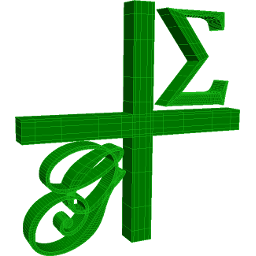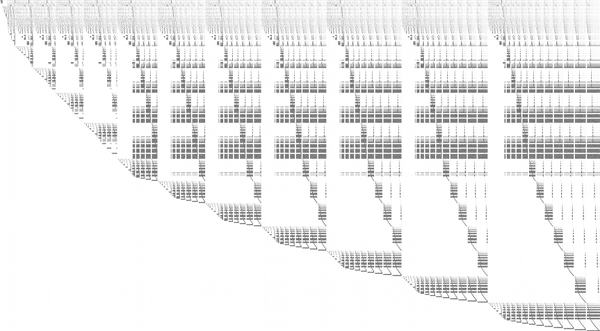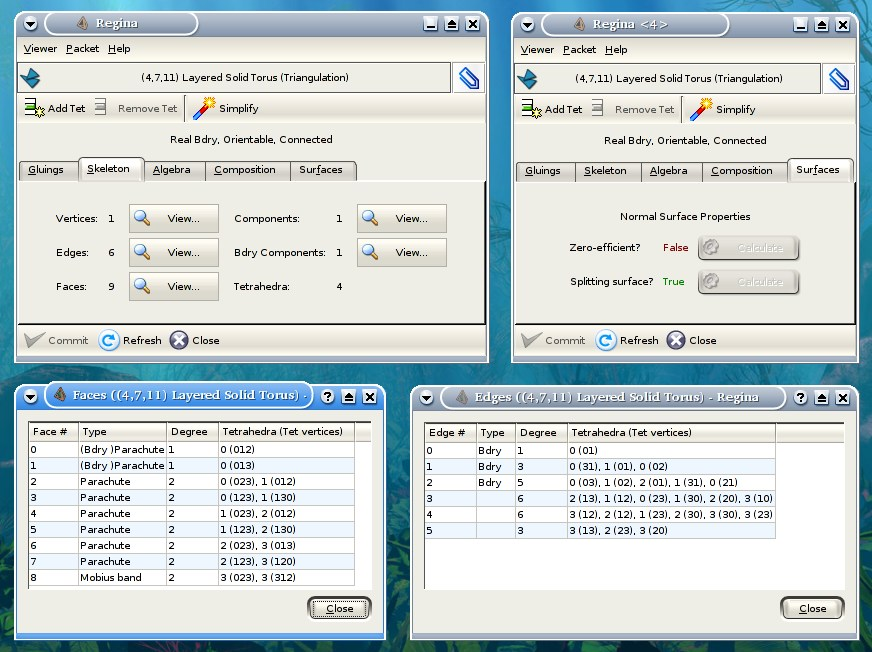## 32 Search Results

### ATLAS

The ATLAS (Automatically Tuned Linear Algebra Software) project is an ongoing research effort focusing on applying empirical techniques in order to provide portable performance. At present, it provides C and Fortran77 interfaces to a portably efficient BLAS implementation, as well as a few routines from LAPACK.

### Axiom

Axiom is a general purpose Computer Algebra system. It is useful for research and development of mathematical algorithms. It defines a strongly typed, mathematically correct type hierarchy. It has a programming language and a built-in compiler.

### CoCoA

CoCoA is a system for Computations in Commutative Algebra. It is able to perform simple and sophisticated operations on multivaraiate polynomials and on various data related to them (ideals, modules, matrices, rational functions). For example, it can readily compute Grobner bases, syzygies and minimal free resolution, intersection, division, the radical of an ideal, the ideal of zero-dimensional schemes, Poincare' series and Hilbert functions, factorization of polynomials, toric ideals. The capabilities of CoCoA and the flexibility of its use are further enhanced by the dedicated high-level programming language. For convenience, the system offers a textual interface, an Emacs mode, and a graphical user interface common to most platforms.

### Fast Artificial Neural Network Library

Fast Artificial Neural Network Library is a neural network library that implements multilayer artificial neural networks in C with support for both fully connected and sparsely connected networks. Cross-platform execution in both fixed and floating point are supported. It includes a framework for easy handling of training data sets. It is easy to use, versatile, well documented, and fast. PHP, C++, .NET, Python, Delphi, Octave, Ruby, Pure Data, and Mathematica bindings are available. A reference manual accompanies the library with examples and recommendations on how to use the library. A graphical user interface is also available for the library.

### FLINT: Fast Library for Number Theory

FLINT is a C library for doing number theory.

### G+Smo

G+Smo (Geometry + Simulation Modules, pronounced "gismo") is a new open-source C++ library that brings together mathematical tools for geometric design and numerical simulation. It is developed mainly by researchers and PhD students. It implements the relatively new paradigm of isogeometric analysis, which suggests the use of a unified framework in the design and analysis pipeline. G+Smo is an object-oriented, cross-platform, template C++ library and follows the generic programming principle, with a focus on both efficiency and ease of use. The library is partitioned into smaller entities, called modules. Examples of available modules include the dimension-independent NURBS module, the data fitting and solid segmentation module, the PDE discretization module and the adaptive spline module, based on hierarchical splines of arbitrary dimension and polynomial degree. The library is licenced under the Mozilla Public License v2.0. It has been developed within the homonym research network supported by the Austrian Science Fund and aims at providing access to high quality, open-source software to the forming isogeometric numerical simulation community and beyond.

More information### GAP

GAP is a system for computational discrete algebra, with particular emphasis on Computational Group Theory. GAP provides a programming language, a library of thousands of functions implementing algebraic algorithms written in the GAP language as well as large data libraries of algebraic objects. GAP is used in research and teaching for studying groups and their representations, rings, vector spaces, algebras, combinatorial structures, and more. GAP is developed by international cooperation. The system, including source, is distributed freely under the terms of the GNU General Public License. You can study and easily modify or extend GAP for your special use. The current version is GAP 4, the older version GAP 3 is still available.

### Global Optimization Toolbox For Maple

Optimization is the science of finding solutions that satisfy complicated constraints and objectives. In engineering, constraints may arise from technical issues. In business, constraints are related to many factors, including cost, time, and staff. The objective of global optimization is to find [numerically] the absolute best solution of highly nonlinear optimization models that may have a number of locally optimal solutions. Global optimization problems can be extremely difficult. Frequently engineers and researchers are forced to settle for solutions that are “good enough” at the expense of extra time, money, and resources, because the best solution has not been found. Using the Global Optimization Toolbox, you can formulate your optimization model easily inside the powerful Maple numeric and symbolic system, and then use world-class Maple numeric solvers to return the best answer, fast! Illustrative references: 1. Pintér, J. D. Global Optimization in Action. Springer Science, 1996, 512 p., ISBN: 978-0-7923-3757-7 Winner of the 2000 INFORMS Computing Society Prize. 2. Pintér, J. D., Linder, D. and Chin, P. Global Optimization Toolbox for Maple: An introduction with illustrative applications. Optimization Methods and Software 21 (2006) (4) 565-582.

### HiFlow³

HiFlow³ is a multi-purpose finite element software providing powerful tools for efficient and accurate solution of a wide range of problems modeled by partial differential equations. Based on object-oriented concepts and the full capabilities of C++ the HiFlow³ project follows a modular and generic approach for building efficient parallel numerical solvers. It provides highly capable modules dealing with the mesh setup, finite element spaces, degrees of freedom, linear algebra routines, numerical solvers, and output data for visualization. Parallelism – as the basis for high performance simulations on modern computing systems – is introduced on two levels: coarse-grained parallelism by means of distributed grids and distributed data structures, and fine-grained parallelism by means of platform-optimized linear algebra back-ends.

### HSL

HSL (formerly the Harwell Subroutine Library) is a collection of ISO Fortran codes for large scale scientific computation, written by members of the Numerical Analysis Group and other experts.

### ILOG CPLEX

ILOG CPLEX is an environment for optimization problems. ILOG CPLEX algorithms can be accessed from the CPLEX Component Libraries as well as the CPLEX Interactive Optimizer, an easy-to-use interactive program. CPLEX provides all the basic features and utilities for using these solvers: sophisticated problem preprocessing; file reading and writing utilities; reporting; messaging control; interactive revision capability; efficient restart from an advanced basis; sensitivity analysis; and an infeasibility finder.

### IML - Integer Matrix Library

IML is a free library of C source code which implements algorithms for computing exact solutions to dense systems of linear equations over the integers. IML is designed to be used with the ATLAS/BLAS library and GMP bignum library. Written in portable C, IML can be used on both 32-bit and 64-bit machines. It can be called from C++.

### LAPACK

LAPACK is written in Fortran77 and provides routines for solving systems of simultaneous linear equations, least-squares solutions of linear systems of equations, eigenvalue problems, and singular value problems. The associated matrix factorizations (LU, Cholesky, QR, SVD, Schur, generalized Schur) are also provided, as are related computations such as reordering of the Schur factorizations and estimating condition numbers. Dense and banded matrices are handled, but not general sparse matrices. In all areas, similar functionality is provided for real and complex matrices, in both single and double precision.

### LattE integrale

LattE (Lattice point Enumeration) is a computer software dedicated to the problems of counting lattice points and integrationinside convex polytopes. LattE contains the first ever implementation of Barvinok's algorithm. The latest version, LattE integrale, has the ability to directly compute integrals of polynomial functions over polytopes and in particular to do exact volume computations. Version 1.6 added the capability of computing the highest coefficients of weighted Ehrhart quasipolynomials.

### LiDIA

LiDIA is a C++ library for computational number theory which provides a collection of highly optimized implementations of various multiprecision data types and time-intensive algorithms.

### LinBox

LinBox is a C++ template library for exact, high-performance linear algebra computation with dense, sparse, and structured matrices over the integers and over finite fields. LinBox has the following top-level functions: solve linear system, matrix rank, determinant, minimal polynomial, characteristic polynomial, Smith normal form and trace. A good collection of finite field and ring implementations is provided, for use with numerous black box matrix storage schemes.

### M4RI

M4RI is a library for fast arithmetic with dense matrices over F2.

More information### Magma

Magma is a large, well-supported software package designed to solve computationally hard problems in algebra, number theory, geometry and combinatorics. It provides a mathematically rigorous environment for computing with algebraic, number-theoretic, combinatoric and geometric objects.

### Matlab

MATLAB is a high-level language and interactive environment that enables you to perform computationally intensive tasks faster than with traditional programming languages such as C, C++, and Fortran.

### Normaliz

Normaliz is a tool for computations in affine monoids, vector configurations, lattice polytopes, and rational cones. Its input data can be specified in terms of a system of generators or vertices or a system of linear homogeneous Diophantine equations, inequalities and congruences or a binomial ideal. Normaliz computes the dual cone of a rational cone (in other words, given generators, Normaliz computes the defining hyperplanes, and vice versa), a placing (or lexicographic) triangulation of a vector configuration (resulting in a triangulation of the cone generated by it), the Hilbert basis of a rational cone, the lattice points of a lattice polytope, the normalization of an affine monoid, the Hilbert (or Ehrhart) series and the Hilbert (or Ehrhart) (quasi) polynomial under a Z-grading (for example, for rational polytopes), NEW: generalized (or weighted) Ehrhart series and Lebesgue integrals of polynomials over rational polytopes via NmzIntegrate, a description of the cone and lattice under consideration by a system of inequalities, equations and congruences

### NTL

NTL is a high-performance, portable C++ library providing data structures and algorithms for manipulating signed, arbitrary length integers, and for vectors, matrices, and polynomials over the integers and over finite fields.

### PLTMG

PLTMG is a package for solving elliptic partial differential equations in general regions of the plane. It is based on continuous piecewise linear triangular finite elements, and features adaptive local mesh refinement, multigraph iteration, and pseudo-arclength continuation options for parameter dependencies. It also provides options for solving several classes of optimal control and obstacle problems. The package includes an initial mesh generator and several graphics packages. Support for the Bank-Holst parallel adaptive meshing strategy is also provided. PLTMG is provided as Fortran (and a little C) source code, in both single and double precision versions. The code has interfaces to X-Windows, MPI, and Michael Holst's OpenGL image viewer SG. The X-Windows, MPI, and SG interfaces require libraries that are NOT provided as part of the PLTMG package.

### PolyBoRi

The core of PolyBoRi is a C++ library, which provides high-level data types for Boolean polynomials and monomials, exponent vectors, as well as for the underlying polynomial rings and subsets of the powerset of the Boolean variables. As a unique approach, binary decision diagrams are used as internal storage type for polynomial structures. On top of this C++-library we provide a Python interface. This allows parsing of complex polynomial systems, as well as sophisticated and extendable strategies for Gröbner base computation. PolyBoRi features a powerful reference implementation for Gröbner basis computation.

More information### Regina

Regina is a suite of mathematical software for 3-manifold topologists. It focuses upon the study of 3-manifold triangulations and includes support for normal surfaces and angle structures.

More information### Risa/Asir

Risa/Asir is a general computer algebra system and also a tool for various computation in mathematics and engineering. The development of Risa/Asir started in 1989 at FUJITSU. Binaries have been freely available since 1994 and now the source code is also free. Currently Kobe distribution is the most active branch of its development. We characterize Risa/Asir as follows: (1) An environment for large scale and efficient polynomial computation. (2) A platform for parallel and distributed computation based on OpenXM protocols.

### Sage

SAGE is a framework for number theory, algebra, and geometry computation. It is open source and freely available under the terms of the GNU General Public License (GPL). SAGE is a Python library with a customized interpreter. It is written in Python, C++, and C (via Pyrex). Python (http://www.python.org) is an open source object-oriented interpreted language, with a large number of libraries, e.g., for numerical analysis, which are available to users of SAGE. Python can also be accessed in library mode from C/C++ programs. SAGE provides an interface to several important open source libraries, including Cremona’s MWRANK library for computing with elliptic curves, the PARI library (pari.math.u-bordeaux.fr) for number theory, Shoup’s number theory library NTL (http://www.shoup.net/ntl/), SINGULAR (http://www.singular.uni-kl.de) for commutative algebra, GAP (http://www.gap-system.org) for group theory and combinatorics, and maxima (http://maxima.sourceforge.net) for symbolic computation and calculus.

More information### SingSurf

An online Java applet for calculation of singular algebraic surfaces.

### SINGULAR

SINGULAR is a Computer Algebra system for polynomial computations in commutative algebra, algebraic geometry, and singularity theory. SINGULAR's main computational objects are ideals and modules over a large variety of baserings. The baserings are polynomial rings over a field (e.g., finite fields, the rationals, floats, algebraic extensions, transcendental extensions), or localizations thereof, or quotient rings with respect to an ideal. SINGULAR features fast and general implementations for computing Groebner and standard bases, including e.g. Buchberger's algorithm and Mora's Tangent Cone algorithm. Furthermore, it provides polynomial factorizations, resultant, characteristic set and gcd computations, syzygy and free-resolution computations, and many more related functionalities. Based on an easy-to-use interactive shell and a C-like programming language, SINGULAR's internal functionality is augmented and user-extendible by libraries written in the SINGULAR programming language. A general and efficient implementation of communication links allows SINGULAR to make its functionality available to other programs.

### Sums over integral points of a polygon

Maple program for computing the sum of values of a polynomial function over the set of integral points of a polygon and the corresponding weighted Ehrhart quasi-polynomial.

### SuperLU

SuperLU is a general purpose library for the direct solution of large, sparse, nonsymmetric systems of linear equations on high performance machines. The library is written in C and is callable from either C or Fortran. The library routines will perform an LU decomposition with partial pivoting and triangular system solves through forward and back substitution. The LU factorization routines can handle non-square matrices but the triangular solves are performed only for square matrices. The matrix columns may be preordered (before factorization) either through library or user supplied routines. This preordering for sparsity is completely separate from the factorization. Working precision iterative refinement subroutines are provided for improved backward stability. Routines are also provided to equilibrate the system, estimate the condition number, calculate the relative backward error, and estimate error bounds for the refined solutions.